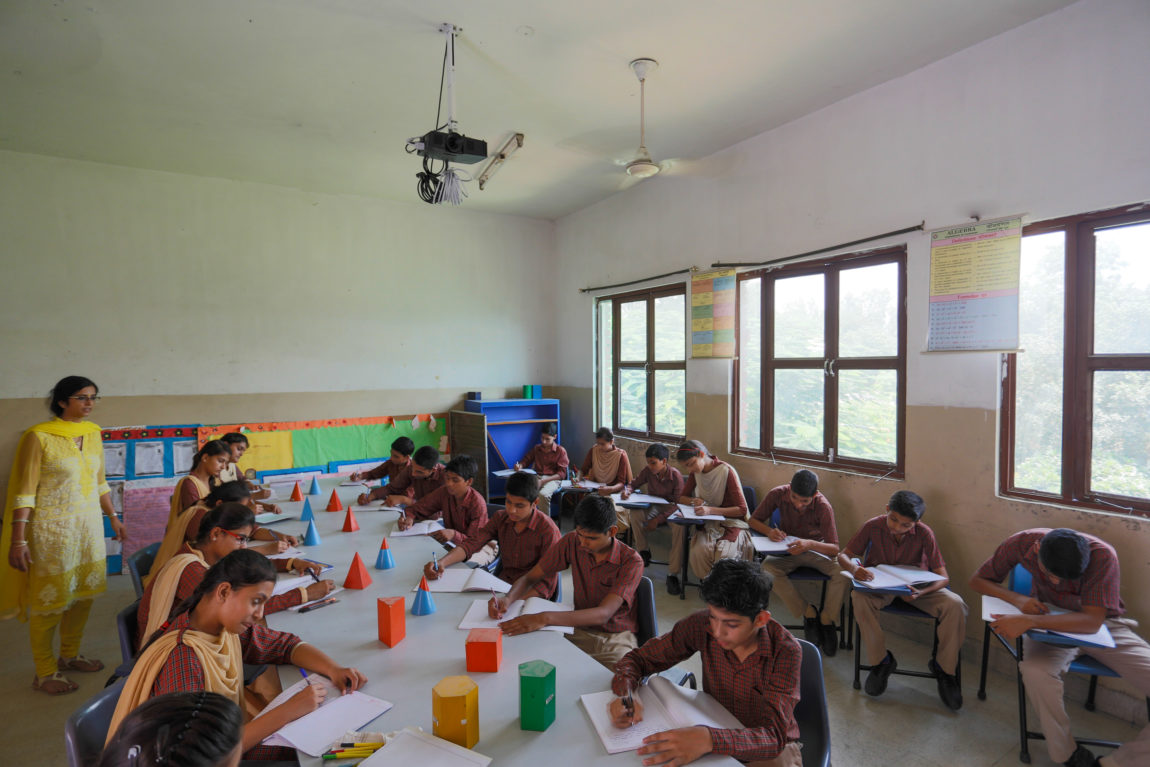The only way to understand mathematics is to do it. Math Lab is a place to learn and explore patterns and ideas. A place where people with varying mathematical proficiency come together to experiment with mathematical ideas and games. While the initial interest is broad based, the eventual engagement is based on their mathematical affinity. In Math Lab mathematical prowess is not judged and it provides an open environment to enjoy mathematics through informal exploration. Students are encouraged to learn all mathematical processes in creative and explorative ways .Some instruments which are used for pedagogical session are:

• Different geometrical models
• Height & distance stand
• Measuring Cylinders
• Abacus Boards
• Circular Plates
• Geographical Boards